## i have one observation with 10 results and i try to scatter the plot but i free something not right

here is the work i did so far, is anyone can help me where is the problem and how can i fix it, thank you very much!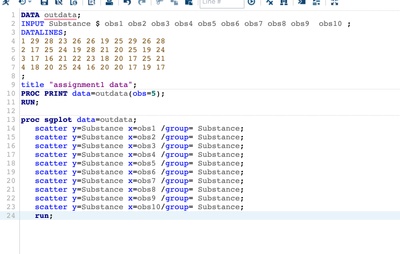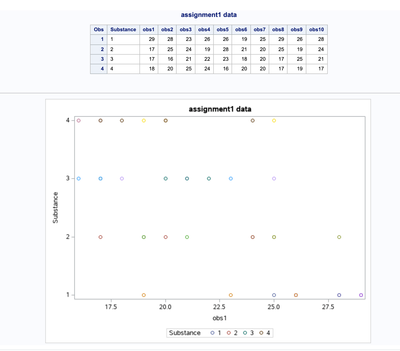1 ACCEPTED SOLUTION

Accepted Solutions

## Re: i have one observation with 10 results and i try to scatter the plot but i free something not ri

Not sure exactly what you are looking for since you are vague. My guess is you want to see the the obs value by Substance in a scatter plot? Do you care about the observation number? Also in the future can you paste code to create the sample data to make it easier for us to test code.

Like @Konu-Tec  said, you probably need to 'melt' the data from wide to narrow. Sometimes called transpose. With SAS you can use PROC TRANPOSE.

``````data outdata;
input Substance \$ obs1-obs4;
datalines;
1 29 28 23 26
2 17 25 24 19
3 17 16 21 22
4 18 20 25 24
;
run;``````

Then transpose/melt the data from wide to narrow using PROC TRANPOSE:

``````proc transpose data=outdata
out=narrowdata(rename=(col1=Value))
name=ObsNumber;
by substance;
run;proc print data=narrowdata;run;``````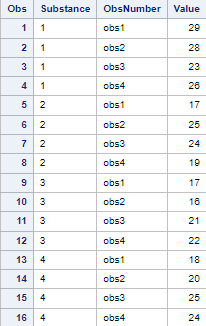Now that the data is in narrow format. Use the SCATTER statement in SGPLOT.

``````proc sgplot data=narrowdata;
scatter x=Substance y=Value;
quit;``````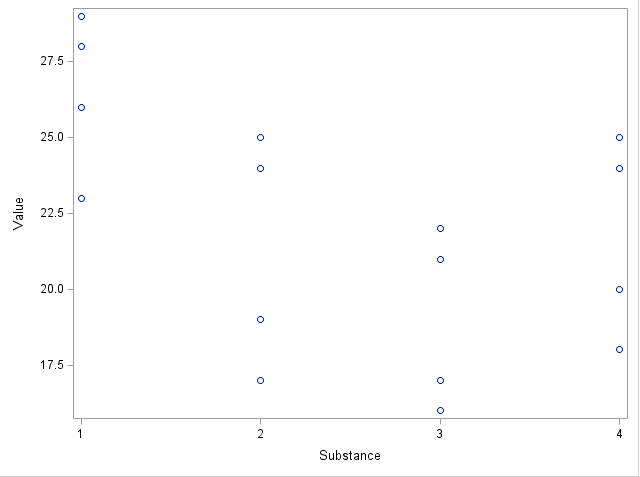I don't like the default image, so you can begin to explore different options and statements in SGPLOT. I'm also not sure exactly what you are looking for, but here is a start:

``````title color=charcoal justify=left height=14pt "Value by Substance";
proc sgplot data=narrowdata;
scatter x=Substance y=Value /
group=Substance
markerattrs=(symbol=circleFilled);
keylegend / noborder title="";
yaxis values=(0 to 35 by 5);
quit;
title;``````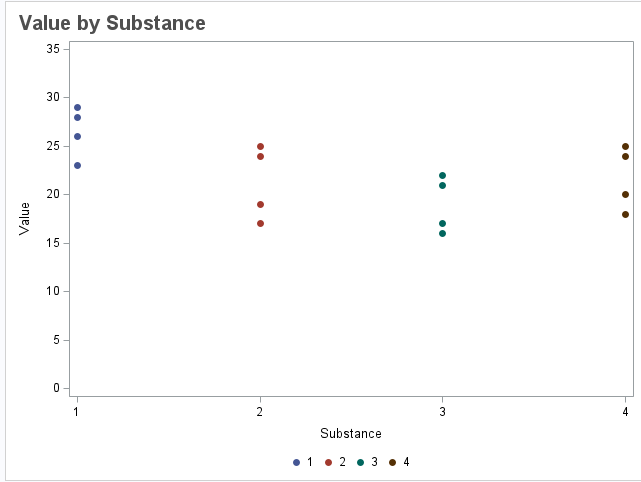- Peter

4 REPLIES 4

## Re: i have one observation with 10 results and i try to scatter the plot but i free something not ri

You might want to melt the data set first or reshape your input

https://pandas.pydata.org/docs/reference/api/pandas.melt.html

## Re: i have one observation with 10 results and i try to scatter the plot but i free something not ri

Not sure exactly what you are looking for since you are vague. My guess is you want to see the the obs value by Substance in a scatter plot? Do you care about the observation number? Also in the future can you paste code to create the sample data to make it easier for us to test code.

Like @Konu-Tec  said, you probably need to 'melt' the data from wide to narrow. Sometimes called transpose. With SAS you can use PROC TRANPOSE.

``````data outdata;
input Substance \$ obs1-obs4;
datalines;
1 29 28 23 26
2 17 25 24 19
3 17 16 21 22
4 18 20 25 24
;
run;``````

Then transpose/melt the data from wide to narrow using PROC TRANPOSE:

``````proc transpose data=outdata
out=narrowdata(rename=(col1=Value))
name=ObsNumber;
by substance;
run;proc print data=narrowdata;run;``````Now that the data is in narrow format. Use the SCATTER statement in SGPLOT.

``````proc sgplot data=narrowdata;
scatter x=Substance y=Value;
quit;``````I don't like the default image, so you can begin to explore different options and statements in SGPLOT. I'm also not sure exactly what you are looking for, but here is a start:

``````title color=charcoal justify=left height=14pt "Value by Substance";
proc sgplot data=narrowdata;
scatter x=Substance y=Value /
group=Substance
markerattrs=(symbol=circleFilled);
keylegend / noborder title="";
yaxis values=(0 to 35 by 5);
quit;
title;``````- Peter

## Re: i have one observation with 10 results and i try to scatter the plot but i free something not ri

thank you so much! so here is another question that if i try to get the ANOVA table, i try the code:

proc anova data = outdata;
class Substance;
model Substance = value;
run;

but there is a error,